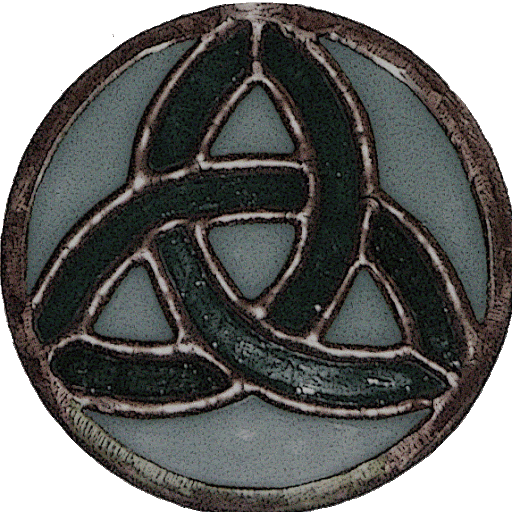Luigi P. - 3 years ago 372
CSS Question

# Text flowing from div to div

I need some divs in which the text automatically flows from one to another. While css regions are still unripe, I found this trick to simulate the effect: https://jsfiddle.net/9o4ebbfL/1/

The trick is valid, but there's an annoying empty space at the beginning of the first div. How can i remove it? Curiously, the original fiddle from which i drew my example (http://jsfiddle.net/natedavisolds/bxzCK/16/) has no additional space, despite the fact that the code is the same.

``````<html lang="it">
<style type="text/css">
.column {
width: 150px;
height: 200px;
overflow:hidden;
float:left;
border: 1px solid #cccccc;
margin: 10px;
}
.column .content {
line-height: 20px;
}
</style>
<script type="text/javascript" src="jquery-1.6.4.js"></script>
<body>

<div id="column1" class="column">
<div class="content">
<p>One plus one is equal to two. Two plus two is equal to four. Three plus three is equal to six. Four plus four is equal to eight. Five plus five is equal to ten. Six plus six is equal to twelve. Seven plus seven is equal to fourteen. Eight plus eight is equal to sixteen. Nine plus nine is equal to eighteen. Ten plus ten is equal to twenty. Eleven plus eleven is equal to twenty-two.</p>
</div>
</div>

<div class="column" data-overflow="#column1">

<script>
\$('.column[data-overflow]').each(function(index) {
var \$this = \$(this),
\$parent = \$(\$this.data('overflow')),
colHeight = \$parent.innerHeight(),
scroll = parseInt(colHeight) * (index + 1),
newHeight = "-=" + scroll + "px";

\$this.html(\$parent.html())
.find('.content').css({ marginTop: newHeight});

});
</script>

</body>
</html>
``````Luke Bajada